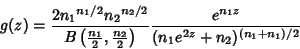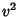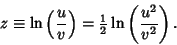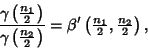## Fisher's z-Distribution(1)

(Kenney and Keeping 1951). This general distribution includes the Chi-Squared Distribution and Student's t-Distribution as special cases. Letandbe Independent Unbiased Estimators of the Variance of a Normally Distributed variate. Define(2)

Then let(3)

so thatis a ratio of Chi-Squared variates(4)

which makes it a ratio of Gamma Distribution variates, which is itself a Beta Prime Distribution variate,(5)

giving(6)

The Mean is(7)

and the Mode is(8)

See also Beta Distribution, Beta Prime Distribution, Chi-Squared Distribution, Gamma Distribution, Normal Distribution, Student's t-Distribution

References

Kenney, J. F. and Keeping, E. S. Mathematics of Statistics, Pt. 2, 2nd ed. Princeton, NJ: Van Nostrand, pp. 180-181, 1951.